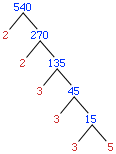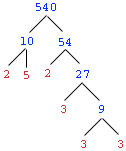My son is in grade seven, he has to do a Factoring Booklet the areas covered are: Prime #'s Composite #'s Rainbow Factoring Finding Multiples (consecutive multiples) Finding GCF of 2-3 numbers uning Rainbow factoring Finding LCM of 2 numbers using consecutive multiples prime factor trees prime factor ladders finding GCF of 2 numbers using Prime number Method Although his text 'Math Power' gives some information, we are at a loss .. Hi Ruth, I hope I can be of some help. A prime number is a positive integer that is only divisible by itself and by 1. Thus 3 is a prime and 13 is a prime but 12 is not a prime since 3 divides 12. I could have said 2 divides 12 or 6 divides 12 or 4 divides 12. Any of these statements shows that 12 is not a prime. A composite number is a positive integer that is not a prime. Thus 12 is a composite number. Here I am at a loss. I don't know the term "Rainbow Factoring". To find the consecutive multiples of a number multiply by 1, then 2, then 3,... Thus, for example the multiples of 12 are 12, 24, 36, 48, 60,... Again I am of no help. To find the least common multiple (LCM) of 2 numbers using consecutive multiples write consecutive multiples of each of them until you find a number that appears in both lists. For example to find the LCM of 12 and 14 write the consecutive multiples 12, 24, 36, 48, 60, 72, 84,... 14, 28, 42, 56, 70, 84, 98,... The first number that appears in both lists is 84 so the LCM of 12 and 14 is 84. Factor Trees are often used to find the prime factors of a number.(For some reasom mathematicians draw trees growing down.) For example to find the prime factors of 54 you might seeI contructed this as follows Divide by 2 as often as possible: 540 = 2270 = 22135. Now try 3 on the 135. 135 = 345 = 3315 = 3335. Since 5 is prime we can stop and deduce that 540 = 223335. You don't need to use prime factors at each step in constructing the tree, but you need to continue down each branch until you arrive at primes. You might have factored 540 using he treeIf you have the prime factorization of two numbers it is relatively straightforward to find the greatest common factor (GCF). For example if you wish to find the GCF of 540 and 126 first find the prime factors, perhaps using the factor tree method. 540 = 223335 126 = 2337               From this factorization you can see that 2 divides both 540 and 126 and 3 divides both of then twice. No other prime number divides both 540 ans 126 so the largest number that divides them both is 233 = 18. Cheers, Penny Go to Math Central